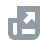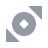شراء العملات الرقمية
الدفع بواسطة
الأسواق
NFT
الموجز
USD

# الأسئلة الأكثر شيوعاًوظائف الحسابالبرنامج التعليمي للمستخدمBinance Fan Tokenأرباح Binanceإيداع\سحب العملات الرقميةشراء العملات الرقمية (النقد الإلزامي\P2P)التداول الفوري والاقتراضي وتداول الصفقات الخارجيةمشتقات العملات الرقميةالموارد الماليةAPIالحمايةشروط استخدام BinanceBinance ConvertNFTVIP
الصفحة الرئيسية
مركز الدعم
الأسئلة الأكثر شيوعاً
مشتقات العملات الرقمية
العملات الرمزية ذات الرافعة المالية
دليل الرموز المميزة ذات الرافعة المالية
Binance Variable Leverage Ratio Reduces Erosion of Net Asset Value (NAV)

# Binance Variable Leverage Ratio Reduces Erosion of Net Asset Value (NAV)

2020-08-21 11:03
What is the erosion of net asset value (NAV)?
When market conditions are volatile, the system frequently rebalances in order to maintain a fixed leverage ratio. This can erode the net asset value (NAV) of leveraged tokens.
Reason:
For leveraged tokens that have a fixed leverage ratio, the rebalancing system starts adjusting your position when the actual leverage ratio begins to deviate from the target. Suppose that a user buys a bullish leveraged token:
• When the price of the underlying asset increases, the actual leverage ratio decreases, so the rebalancing mechanism increases your position. At this time, if the price of the underlying asset begins to fall, it triggers the system to perform a "top position addition". This causes further losses as the price of the asset goes down, which leads to greater losses of the user's total funds, thereby causing the erosion of net asset value (NAV).
• When the price of the underlying asset falls, the actual leverage ratio increases, so the rebalancing mechanism reduces your position to lock-in the loss. At this time, if the price of the underlying asset begins to increase, it triggers the system to perform a "bottom position reduction". Even if the price recovers, the losses of the user's total funds cannot be recovered, thereby causing the erosion of net asset value (NAV).
Example:
Suppose that a bullish leveraged token has a fixed leverage ratio of 3x. These tokens are indicated using LT 3X as follows:
• A basket position consists of 1000 BTC/USDT perpetual contracts at a price of 9000 USDT, so the total position is worth 9 million USDT;
• The number of issued leverage tokens is 300,000 LT 3X with a net asset value (NAV) of 10 USDT, so the user's funds total 3 million USDT;
• Current actual leverage ratio = Total position / Net asset value (NAV) = 3, which is equal to the target leverage ratio.
On Day 1, the user purchased 900 LT 3X tokens. If the price of a BTC/USDT perpetual contract goes +10% on Day 1, -10% on Day 2, and then +10% on Day 3, we can see how the net asset value (NAV) of the user's funds erodes:
Day 1:
• BTC/USDT perpetual contract price = 9000 USDT;
• LT 3X net asset value (NAV) = 10 USDT;
• Actual leverage ratio = 3;
• User's total assets = 900 × 10 = 9000 USDT.
Day 2:
Suppose that the price of a BTC/USDT perpetual contract increases by 10%:
• BTC/USDT perpetual contract price = 9000 × (1 + 10%) = 9900 USDT;
• Total position = 9900 × 1000 = 9.9 million USDT, with a profit of 900,000 USDT;
• Accordingly, the user's funds also increase by 900,000 USDT: 3 million + 900,000 = 3.9 million USDT;
• Net asset value (NAV) = Total funds / Issued tokens = 3.9 million / 300,000 = 13, which is an increase of 30%;
• At this time, the actual leverage ratio = 9.9 million / 3.9 million = 2.54;
• User's total assets = 900 × 13 = 11700 USDT.
Rebalancing triggered: Here, the actual leverage ratio is not equal to the target leverage ratio of 3, which triggers the rebalancing mechanism:
• The system buys more of the underlying asset to add to the user's total position and increases the "base assets" of the user's leveraged token funds. If the price of the underlying asset continues to increase, the user will make even more profit. However, if the market begins to fall, this is equivalent to a "top position increase" - the same fall in price results in even greater losses;
• The system adds to the user's total position by buying 182 BTC/USDT perpetual contracts;
• Total position = 9900 × (1000 + 182) = 11.7 million USDT;
• Actual leverage ratio = 11.7 million / 3.9 million = 3. The position is adjusted to ensure that the actual leverage ratio stays equal to the target leverage ratio.
Day 3:
Suppose that the price of a BTC/USDT perpetual contract falls 10%:
• BTC/USDT perpetual contract price = 9900 × (1 - 10%) = 8910 USDT;
• Total position = 8910 × (1000 + 182) = 10.53 million USDT;
• The loss to the user's total position is 11.7 million - 10.53 million = 1.17 million USDT;
• Accordingly, the user's total funds also incur a loss of 1.17 million: 3.9 million - 1.17 million = 2.73 million USDT;
• Here, the user's total funds incur an additional loss of the 182 USDT top position increase:
• LT 3X net asset value (NAV) = 2.73 million / 300,000 = 9.1 USDT;
• User's total assets = 900 × 9.1 = 8190 USDT.
Summary:
• The price of the underlying asset rose by 10%, but the user's total funds only increased by 1000 USDT. The rebalancing mechanism added a top position increase of 182 USDT. Then, the price of the underlying asset decreased by 10% and the user's total funds had to bear the loss of the 182 USDT, thereby reducing the net asset value (NAV) of the user's account.
• When the price of the underlying asset first fell by 10%, and then rose by 10%, the rebalancing mechanism reduced the user's total position using a bottom position reduction, which realized the loss by reducing the user's position. By the time the price of the underlying asset increased by 10%, the user's total position had already been reduced, so only a portion of the loss was able to be recovered. In the end, only a portion of the user's total funds was recovered, so the net asset value (NAV) of the user's account decreased.
If market conditions continue to fluctuate, rising or falling by 10% each day, the final net asset value (NAV) of the LT 3X token and the user's assets will be as shown below:
Therefore, leveraged tokens that use a fixed leverage ratio are subject to high erosion of net asset value (NAV) when the market is volatile.
Binance's variable Leverage Ratio Reduce Erosion of Net Asset Value (NAV)
To protect users' assets and create a better user experience, Binance uses a variable leverage ratio which is maintained between 1.25x and 4x. When rebalancing, Binance adjusts the actual leverage ratio to a level within the target range as determined by the algorithm.
Example:
• Suppose that a basket position for the Binance Leveraged Token BTCUP is 1000 BTC/USDT perpetual contracts priced at 9000 USDT, which makes the user's total position worth 9 million USDT;
• The number of issued leverage tokens is 450,000 BTCUP with a net asset value (NAV) of 10 USDT, so the user's funds total of 4.5 million USDT;
• Current leverage ratio = Total position / Net asset value (NAV) = 2, which is within the target range of 1.25x - 4x.
On Day 1, the user purchased 900 BTCUP. If the price of a BTC/USDT perpetual contract goes +10%, -10%, and then +10% on Days 1, 2, and 3, respectively;
Day 1:
• BTC/USDT perpetual contract price = 9000 USDT;
• LT 3X net asset value (NAV) = 10 USDT;
• Actual leverage ratio = 2;
• User's total assets = 900 × 10 = 9000 USDT.
Day 2:
Suppose that the price of a BTC/USDT perpetual contract increases by 10%:
• BTC/USDT perpetual contract price = 9000 × (1 + 10%) = 9900 USDT;
• Total position = 9900 × 1000 = 9.9 million USDT, with a profit of 900,000 USDT;
• Accordingly, the user's funds also increase by 900,000 USDT: 4.5 million + 900,000 = 5.4 million USDT;
• Net asset value (NAV) = Total funds / Issued tokens = 5.4 million / 450,000 = 12, which is an increase of 20%;
• At this time, the actual leverage ratio = 9.9 million / 5.4 million = 1.83;
• User's total assets = 900 × 12 = 10,800 USDT;
Day 3:
Suppose that the price of a BTC/USDT perpetual contract falls 10%:
• BTC/USDT perpetual contract price = 9900 × (1 - 10%) = 8910 USDT;
• Total position = 8910 × 1000 = 8.91 million USDT;
• Loss to total position is 9.9 million - 8.91 million = 990,000 USDT;
• Correspondingly, the user's total funds also incur a loss of 990,000: 5.4 million - 990,000 = 4.41 million USDT;
• BTCUP net asset value (NAV) = 4.41 million / 450,000 = 9.8 USDT;
• User's total assets = 900 × 9.8 = 8820 USDT.
If market conditions continue to fluctuate, rising or falling by 10% each day, the price of a BTC/USDT perpetual contract, the price of the BTCUP leveraged token, the actual leverage ratio, and the user's assets will all change over time as shown below:
Summary:
Since Binance uses a variable leverage ratio, the erosion of the user's net asset value (NAV) due to frequent position adjustments in volatile market conditions is greatly reduced.